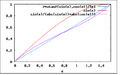# File:AngleApproximationQuality.png

Graph showing that $\frac{y}{|x|+|y|}$ gives a (much) better estimate of the angle than a sine ($\frac{y}{\sqrt{x^2+y^2}}$). Note that the blue line is the ideal line.
current12:05, 17 April 2009412 × 257 (4 KB)Jaspervdg (Talk | contribs)Graph showing that $\frac{y}{|x|+|y|}$ gives a (much) better estimate of the angle than a sine ($\frac{y}{\sqrt{x^2+y^2}}$).